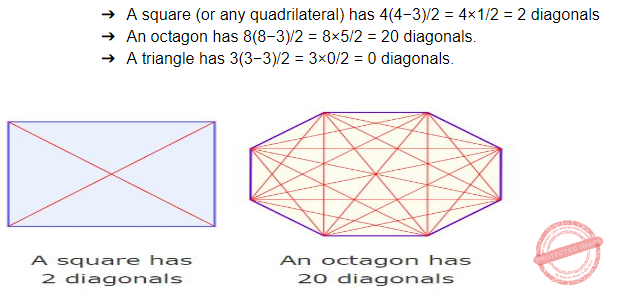## Geometry

 Question 1
How many diagonals can be drawn by joining the angular points of an octagon?How many diagonals can be drawn by joining the angular points of an octagon?
 A 14 B 20 C 21 D 28
Aptitude       Geometry       ISRO CS 2013       Video-Explanation
Question 1 Explanation:
→A polygon's diagonals are line segments from one corner to another (but not the edges).
→The number of diagonals of an n-sided polygon is: n(n − 3) / 2.
→Examples:Question 2
The conic section that is obtained when a right circular cone is cut through a plane that is parallel to the side of the cone is called
 A parabola B hyperbola C circle D ellipse
Engineering-Mathematics       Geometry       ISRO CS 2014       Video-Explanation
Question 2 Explanation:There are 2 questions to complete.

Register Now